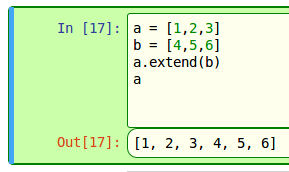From two arrays to one arrays

Hello!
if I would like to obtain from two array:
A = [a b c]
B = [d e f]
the following array
C = [a b c d e f]
there is a istruction to unite the arrays A and B?

Good life!

Fede

I didn’t tell you that I use StarBasic

https://help.libreoffice.org/Calc/Operators_in_Calc

See tilde (~) operator. E.g.: =SUM(A1:A3~C4:C6)

Cannot be used in Data->Validity.

EDIT: Since you need a BASIC function, you may use this:

Function UnionArrays (Arr1, Arr2)
' Expects two 1-dimensional arrays
Dim result
result = Arr1
ReDim Preserve result(UBound(Arr1)+UBound(Arr2)-LBound(Arr2)+1)
Dim i As Integer
i = UBound(Arr1)+1
Dim n As Integer
For n=LBound(Arr2) To UBound(Arr2)
result(i) = Arr2(n)
i = i+1
Next n
UnionArrays = result
End Function

I’m sorry,
I didn’t tell you that I use Starbasic

Thank You Mike :D,
Good Life

Hallo

It’s easy as pie!Now that I’m thinking about it, OP does make 3 arrays (or lists, as Python, pictured above, calls them) which is using same syntax some languages use, and I don’t remember seeing arrays made that way in Calc. I could be wrong, though. Python does make many things much easier than any other language AFAIK.

I didn’t tell you that I use StarBasic
sorry

Hi

a = array("a","b","c")
b = array("d","e","f")

c = split(join(a, ",") & "," & join(b, ","), ",")

Regards

works only with strings inside array, and maybe partielly with integers or floats…
python works with any objects inside lists